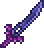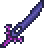•类型 \l!--\n --\g\{\{#vardefine:_typepage\!\{\{#or:\{\{l10n\!item_infobox\!typepage:@@@@\}\}\!\{\{tr\!\{\{ucfirst:@@@@\}\}\!link\ey\}\}\}\}\}\}\l!--\_\n --\g\{\{#vardefine:_typetext\!\{\{#or:\{\{l10n\!item_infobox\!type:@@@@\}\}\!\{\{tr\!\{\{ucfirst:@@@@\}\}\}\}\}\}\}\}\l!--\_\n --\g\{\{nowrap\!class\etag\!\{\{ifexists\!\{\{#var:_typepage\}\}\!\(\(\{\{#var:_typepage\}\}\!\{\{#var:_typetext\}\}\)\)\!\{\{#var:_typetext\}\}\}\}\}\}\l!--\n --\g\l!--\n --\g\{\{#vardefine:_typepage\!\{\{#or:\{\{l10n\!item_infobox\!typepage:@@@@\}\}\!\{\{tr\!\{\{ucfirst:@@@@\}\}\!link\ey\}\}\}\}\}\}\l!--\_\n --\g\{\{#vardefine:_typetext\!\{\{#or:\{\{l10n\!item_infobox\!type:@@@@\}\}\!\{\{tr\!\{\{ucfirst:@@@@\}\}\}\}\}\}\}\}\l!--\_\n --\g\{\{nowrap\!class\etag\!\{\{ifexists\!\{\{#var:_typepage\}\}\!\(\(\{\{#var:_typepage\}\}\!\{\{#var:_typetext\}\}\)\)\!\{\{#var:_typetext\}\}\}\}\}\}\l!--\n --\g 42 (近战) 4.5（普通击退力） 4% 21（快速度） / 27（普通速度）4 需要 1 份

使用 \{\{#lstmap:\$soundfiles\$\!,\!%%%%\!\l!--\_print\_sounds\n --\g\lspan\_style\e"margin-left:2px;"\g\{\{sound\!\!\{\{trim\!%%%%\}\}\}\}\l/span\g\l!--\n --\g\!\}\}

!!Error: （请输入有效的值。参见Template:Modifier。）

## 制作

total: 2 row(s)

### 制作树

 × 20(+) × 60( 地狱 ) × 30 40  () × 30 40  () × 12 × 12 15  ()() × 10 × 10////或/total: 2 row(s)

## 备注

• 因为永夜刃需要来自地牢村正，因此它只在骷髅王被打败之后才可获得。
• 在中，几乎总是会在地牢守卫生成的深度以上生成一个锁住的金箱，并且其中总会有一把村正。因此，有可能在打败骷髅王之前就得到永夜刃。

## 花絮

• 1.1 更新以前，永夜刃是游戏中最强大的剑，尽管此地位现在已被推翻了。尽管如此，它仍然是最强的困难模式之前的剑。\{\{comma/item\!\l!--\n --\g\{\{#var:_s_bi_start\}\}\l!--\_bold/italic\_opening\_tags\n --\g\{\{@@@@\_version\!\{\{#var:_s_mode\}\}\!short\e\{\{#var:_s_short\}\}\!nl\e\{\{#var:_s_nl\}\}\!small\e\{\{#var:_s_small\}\}\}\}\l!--\n --\g\{\{#var:_s_bi_end\}\}\l!--\_bold/italic\_closing\_tags\n --\g\}\}上除外，在其中晶光刃在困难模式之前已经可用。
• 永夜刃是唯一一种在祭坛上制作又不是 Boss 召唤物品的物品。
• 用于制作它的四把剑各自代表了困难模式之前的一种主要的困难生物群落：草剑代表的是丛林，村正是地牢，炽焰巨剑是地狱，魔光剑/血腥屠刀代表的是邪恶生物群落
• 永夜刃在现实中也有相应的玩具，由 Jazwares 制造。
• 它在《地牢守护者 2》中作为 Phantom's Edge 出现。
• 无论用的是哪个制作配方，因为永夜刃散发出来的颗粒的关系，它都像是腐化主题的剑。
• 此剑的微粒包括了制作此剑所用的全部剑：村正（正十字）、炽焰巨剑（斜十字）、草剑（小泡泡）、和魔光剑/血腥屠刀（大泡泡）。

## 历史

• 电脑版 1.4.1
• 使用时间由 27 降低至 21。
• 现在能自动挥舞了。
• 主机版 1.02
• 现在用于原版永夜刃的制作。
• 血腥屠刀现在可以用来在制作配方中替代魔光剑。
• 出售价值由 54 提升至 18
• 主机正式版：伴随着电脑版 1.0.6 的相应外观和机制引入。
• 移动版 1.2.11212
• 现在用于原版永夜刃的制作。
• 血腥屠刀现在可以用来在制作配方中替代魔光剑。
• 出售价值由 54 提升至 18
• 移动正式版：伴随着电脑版 1.0.6 的相应外观和机制引入。
• 3DS正式版：和机制、配方、外观一道由电脑版 1.2 引入。

## 参考

1. Terraria-Based Toys Now Available! 2014 年 10 月 30 日